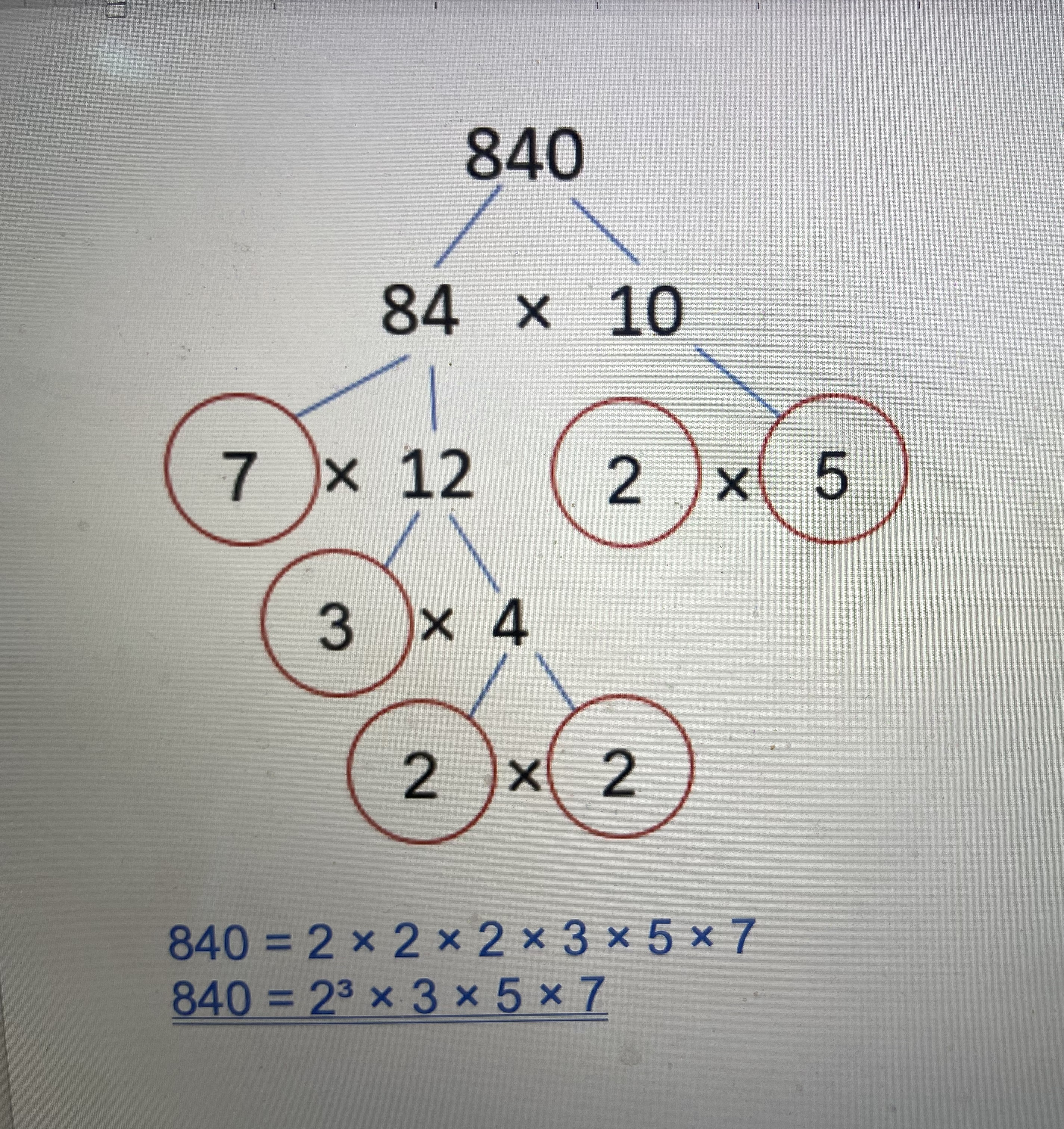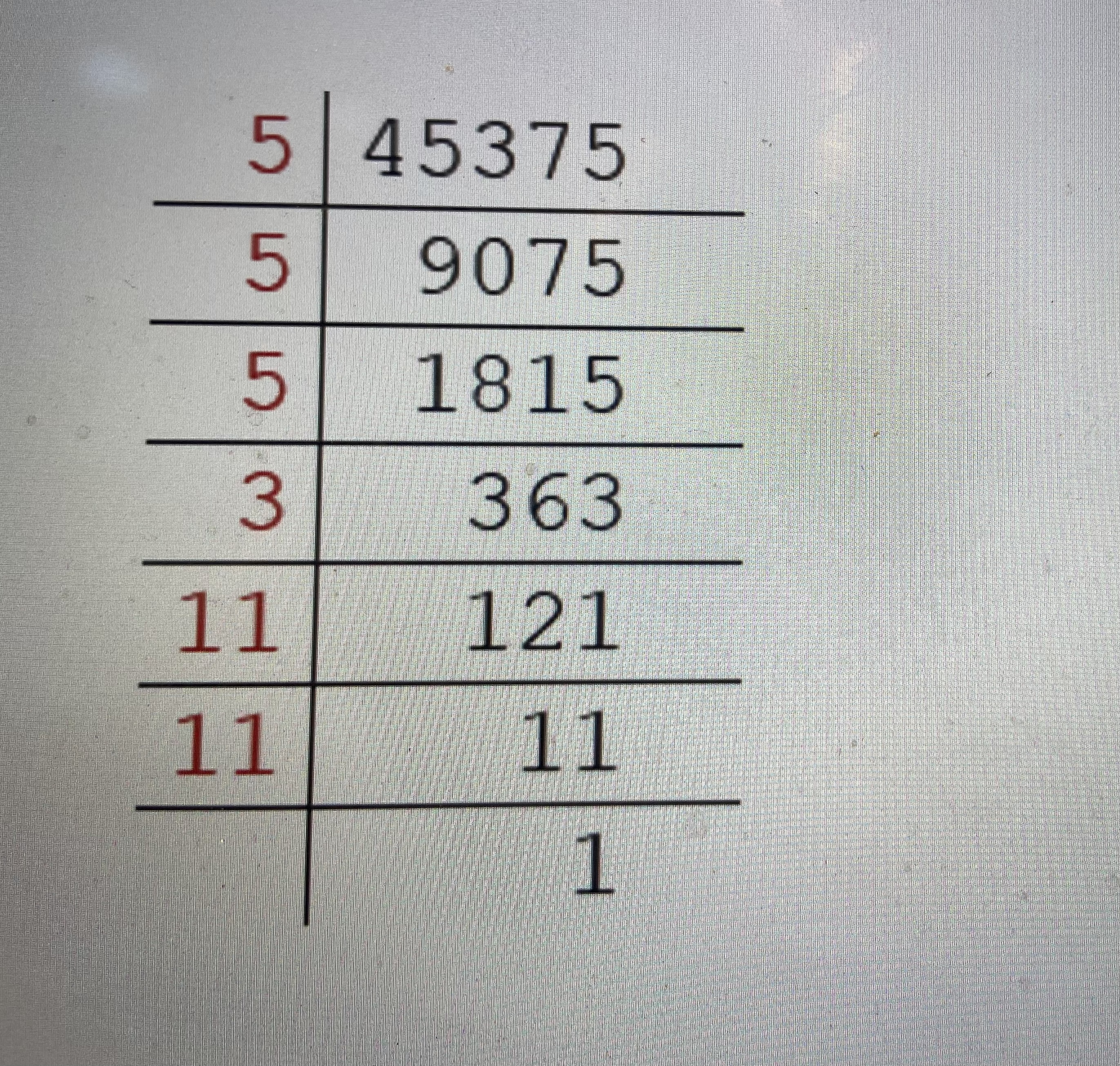• Nursery
• Pre Prep
• Upper School

# Of Prime Importance…

One of the concepts the children are introduced to in Form 5 or 6 is that of prime numbers.

(There is a glossary of terms at the bottom of this blog).

So, the first few prime numbers are 2, 3, 5, 7 and 11

At Cargilfield, children usually start to work with prime numbers in Form 5 or 6. Once they have been introduced to them, they will learn to express other numbers as a product of their prime factors, e.g. 100 = 2 × 2 × 5 × 5 or 2² × 5²

One method of doing this is to use the factor tree, sometimes called the ‘cherry tree’. A number is gradually broken up until the prime numbers at its root are found and circled.

For example, express 840 as a product of its prime factors:840 = 2 × 2 × 2 × 3 × 5 × 7

840 = 2³ × 3 × 5 × 7

Another method is called the ‘ladder’. This method is useful for numbers that do not as easily lend themselves to being broken up using mental arithmetic.

The way this works is a number is divided by prime numbers only until a final quotient of 1 is reached.

For example, express 45375 as a product of its prime factors:45375 = 3 × 5 × 5 × 5 × 11 × 11

45375 = 3 × 5³ × 11²

Knowing the product of prime factors of a number has a wide range of applications.

Here are some ways the children might use the product of prime factors:

Given that 784 = 2⁴ × 7² and 1638 = 2 × 32 × 7 × 13, answer the following questions.

• a) Is 1638 a multiple of 9? Yes, because 3×3 can be found in the product of prime factors of 1638
• b) What is the largest odd factor of 784? Multiply the odd prime factors only: 7 × 7 = 49
• c) What is the highest common factor of 784 and 1638? 2 × 7 is common to both numbers, therefore 14 is the highest common factor.
• d) Which of 784 and 1638 is a perfect square? 784, because it has an even number of each of its primes.
• e) Work out the square root of 784. Separate the product of prime factors into two equal groups and multiply out one of the groups: 2 × 2 × 7 = 28
• f) What is the lowest common multiple of 784 and 1638? Multiply out the higher quantity of each prime: 2⁴ × 3² × 7² × 13 = 91 728

Whatever the level of application of product of prime factors, one essential ingredient to developing the children’s confidence is a fluent knowledge of times tables, not just the multiplication (7 × 9 = 63) but also the division too (63 ÷ 9 = 7).

Glossary of Terms

factor - A number that divides another number exactly. E.g. 6 is a factor of 18

multiple - A number which is part of another number's times table. E.g. 42 is a multiple of 7

prime number - A number which has exactly two factors. The number one and itself.

highest common factor - It is the largest factor common to a set of numbers. E.g. The HCF of 12 and 18 is 6

lowest common multiple - It is the smallest multiple common to a set of numbers. E.g. The LCM of 4 and 6 is 12

quotient - a result obtained by dividing one quantity by another.

Posted on

# Of Prime Importance…

One of the concepts the children are introduced to in Form 5 or 6 is that of prime numbers.

(There is a glossary of terms at the bottom of this blog).

So, the first few prime numbers are 2, 3, 5, 7 and 11

At Cargilfield, children usually start to work with prime numbers in Form 5 or 6. Once they have been introduced to them, they will learn to express other numbers as a product of their prime factors, e.g. 100 = 2 × 2 × 5 × 5 or 2² × 5²

One method of doing this is to use the factor tree, sometimes called the ‘cherry tree’. A number is gradually broken up until the prime numbers at its root are found and circled.

For example, express 840 as a product of its prime factors:840 = 2 × 2 × 2 × 3 × 5 × 7

840 = 2³ × 3 × 5 × 7

Another method is called the ‘ladder’. This method is useful for numbers that do not as easily lend themselves to being broken up using mental arithmetic.

The way this works is a number is divided by prime numbers only until a final quotient of 1 is reached.

For example, express 45375 as a product of its prime factors:45375 = 3 × 5 × 5 × 5 × 11 × 11

45375 = 3 × 5³ × 11²

Knowing the product of prime factors of a number has a wide range of applications.

Here are some ways the children might use the product of prime factors:

Given that 784 = 2⁴ × 7² and 1638 = 2 × 32 × 7 × 13, answer the following questions.

• a) Is 1638 a multiple of 9? Yes, because 3×3 can be found in the product of prime factors of 1638
• b) What is the largest odd factor of 784? Multiply the odd prime factors only: 7 × 7 = 49
• c) What is the highest common factor of 784 and 1638? 2 × 7 is common to both numbers, therefore 14 is the highest common factor.
• d) Which of 784 and 1638 is a perfect square? 784, because it has an even number of each of its primes.
• e) Work out the square root of 784. Separate the product of prime factors into two equal groups and multiply out one of the groups: 2 × 2 × 7 = 28
• f) What is the lowest common multiple of 784 and 1638? Multiply out the higher quantity of each prime: 2⁴ × 3² × 7² × 13 = 91 728

Whatever the level of application of product of prime factors, one essential ingredient to developing the children’s confidence is a fluent knowledge of times tables, not just the multiplication (7 × 9 = 63) but also the division too (63 ÷ 9 = 7).

Glossary of Terms

factor - A number that divides another number exactly. E.g. 6 is a factor of 18

multiple - A number which is part of another number's times table. E.g. 42 is a multiple of 7

prime number - A number which has exactly two factors. The number one and itself.

highest common factor - It is the largest factor common to a set of numbers. E.g. The HCF of 12 and 18 is 6

lowest common multiple - It is the smallest multiple common to a set of numbers. E.g. The LCM of 4 and 6 is 12

quotient - a result obtained by dividing one quantity by another.

Posted on

## Cargilfield where everyday is an adventureWelcome to Cargilfield! We hope this short film gives you a glimpse of what life is like for the girls and boys at our school. We would love to welcome you in person to tour Cargilfield and explain more fully exactly what makes a Cargilfield education so special and so different. Please get in touch with Fiona Craig, our Registrar if you would like to find out more; her email address is [email protected] or you can telephone her on 0131 336 2207.

Don't Show Again